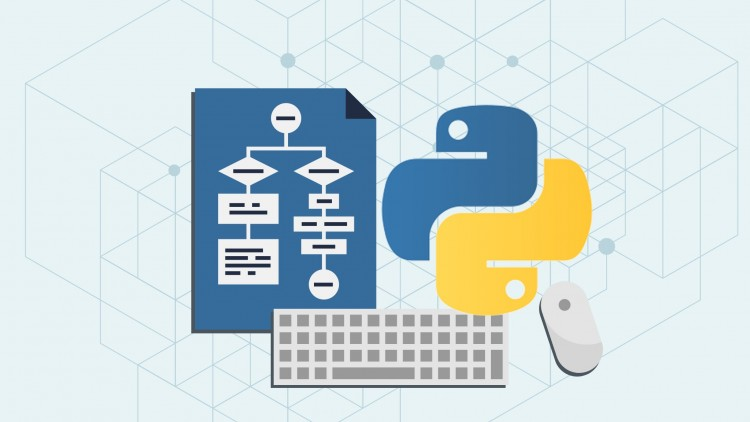# Interesting Method to Generate Binary Numbers from 1 to nInteresting Method to Generate Binary Numbers from 1 to n Given a number n, write a function that generates and prints all binary numbers

Method to Generate Binary Numbers

Given a number n, write a function that generates and prints all binary numbers with decimal values from 1 to n.

### Examples:

```Input: n = 2
Output: 1, 10

Input: n = 5
Output: 1, 10, 11, 100, 101
```

A simple method is to run a loop from 1 to n, call decimal to binary inside the loop.Following is an interesting method that uses queue data structure to print binary numbers.

1) Create an empty queue of strings
2) Enqueue the first binary number “1” to queue.
3) Now run a loop for generating and printing n binary numbers.
a) Dequeue and Print the front of queue.
b) Append “0” at the end of front item and enqueue it.
c) Append “1” at the end of front item and enqueue it.

#### Python Programming Method to Generate Binary Numbers

``````# Python program to generate binary numbers from
# 1 to n

# This function uses queu data structure to print binary numbers
def generatePrintBinary(n):

# Create an empty queue
from Queue import Queue
q = Queue()

# Enqueu the first binary number
q.put("1")

# This loop is like BFS of a tree with 1 as root
# 0 as left child and 1 as right child and so on
while(n>0):
n-= 1
# Print the front of queue
s1 = q.get()
print s1

s2 = s1 # Store s1 before changing it

# Append "0" to s1 and enqueue it
q.put(s1+"0")

# Append "1" to s2 and enqueue it. Note that s2
# contains the previous front
q.put(s2+"1")

# Driver program to test above function
n = 10
generatePrintBinary(n)
``````

### Output:

```1
10
11
100
101
110
111
1000
1001
1010```
READ  How to efficiently implement k stacks in a single array#### Venkatesan Prabu

Wikitechy Founder, Author, International Speaker, and Job Consultant. My role as the CEO of Wikitechy, I help businesses build their next generation digital platforms and help with their product innovation and growth strategy. I'm a frequent speaker at tech conferences and events.

X